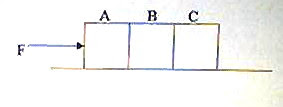## Saturday, July 10, 2010

### Multiple Choice Questions Involving Friction

Any intelligent fool can make things bigger and more complex…

It takes a touch of genius and a lot of courage to move in the opposite direction.

Albert Einstein

Here are two questions (MCQ) on friction, which may easily tempt you to answer incorrectly:(1) Three identical wooden blocks A, B and C, each of mass 1 kg, are placed on a rough horizontal surface as shown. A horizontal force F acts on the block A and the system of masses moves with an acceleration of 2 ms–2. If the coefficient of friction between the blocks and the horizontal surface is 0.3 and the gravitational acceleration is 10 ms–2, the force exerted by block A on block B is

(a) 2 N

(b) 4 N

(c) 6 N

(d) 8N

(e) 10 N

The block A pushes the blocks B and C and produces an acceleration in them. But when the blocks A and B move, an opposing frictional force Ff is produced. The opposing frictional force is given by

Ff = μmg where μ is the coefficient of friction, m is the total mass of blocks A and B and g is the gravitational acceleration.

Therefore, Ff = 0.3×2×10 = 6 N.

The resultant force acting on block B is FB Ff where FB is the force exerted by block A on block B. Therefore, we have

FB Ff = ma = 2×2 since the resultant force moves blocks A and B with an acceleration of 2 ms–2.

Therefore, FB – 6 = 4 so that FB = 10 N.(2) A block of mass M slides down with uniform velocity from the top A to the bottom B of a rough inclined plane of angle θ and height H. If the coefficient of kinetic friction between the block and the plane is μk, the work done against friction during the motion of the block is

(a) MgH sin θ

(b) μkMgH sin θ

(c) μkMgH/ sin θ

(d) μkMgH

(e) MgH

When the block reaches the bottom of the incline, the gravitational potential energy of the block decreases by MgH. If the plane were smooth, the kinetic energy of the block would have increased by an equal amount. Since the kinetic energy of the block remains unchanged, it follows that the loss in the gravitational potential energy is equal to the work done against friction [Option (e)].

Now see two posts in this section here.